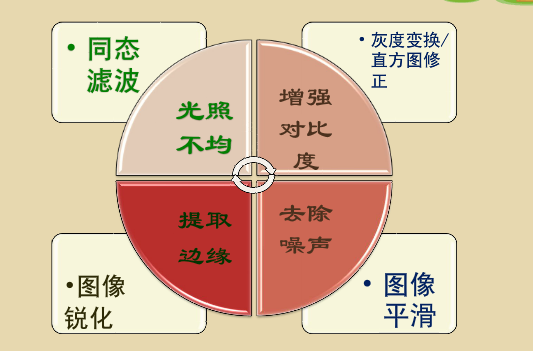# MATLAB图像处理_同态滤波

S(x,y)---->Log---->DFT---->频域滤波---->IDFT---->Exp---->T(x,y)function I3 = test_tontai(I)

I=double(rgb2gray(I));
[M,N]=size(I);
rL=0.5;
rH=4.7;%可根据需要效果调整参数
c=2;
d0=10;
I1=log(I+1);%取对数
FI=fft2(I1);%傅里叶变换
n1=floor(M/2);
n2=floor(N/2);
for i=1:M
for j=1:N
D(i,j)=((i-n1).^2+(j-n2).^2);
H(i,j)=(rH-rL).*(exp(c*(-D(i,j)./(d0^2))))+rL;%高斯同态滤波
end
end
I2=ifft2(H.*FI);%傅里叶逆变换
I3=real(exp(I2));
subplot(122),imshow(I3,[]);title('同态滤波增强后');11-121557
05-172万+
02-251万+
08-191万+
02-233万+
04-289045
04-141844
11-302969
12-154100
03-17166
03-17254
05-074万+
12-12757
05-153142
04-061270
03-252626
10-173万+

### “相关推荐”对你有帮助么？

•非常没帮助
•没帮助
•一般
•有帮助
•非常有帮助被折叠的  条评论 为什么被折叠?到【灌水乐园】发言¥2 ¥4 ¥6 ¥10 ¥20余额支付 (余额：-- )扫码支付获取中扫码支付点击重新获取扫码支付1.余额是钱包充值的虚拟货币，按照1:1的比例进行支付金额的抵扣。
2.余额无法直接购买下载，可以购买VIP、C币套餐、付费专栏及课程。余额充值